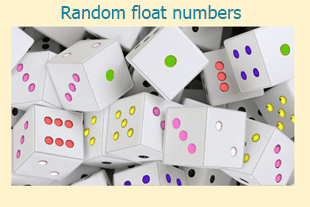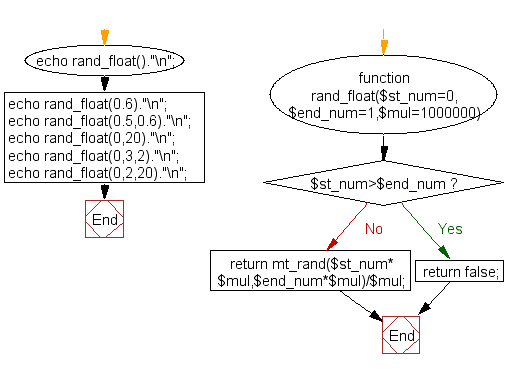﻿ PHP Math Exercise: Get random float numbers - w3resource# PHP Math Exercises: Get random float numbers

## PHP math: Exercise-10 with Solution

Write a PHP function to get random float numbers.

Pictorial Presentation:Sample Solution:

PHP Code:

``````<?php
function rand_float(\$st_num=0,\$end_num=1,\$mul=1000000)
{
if (\$st_num>\$end_num) return false;
return mt_rand(\$st_num*\$mul,\$end_num*\$mul)/\$mul;
}
echo rand_float()."\n";
echo rand_float(0.6)."\n";
echo rand_float(0.5,0.6)."\n";
echo rand_float(0,20)."\n";
echo rand_float(0,3,2)."\n";
echo rand_float(0,2,20)."\n";
?>
```
```

Sample Output:

```0.613314
0.886207
0.57955
17.570268
1.5
1.6
```

Flowchart :PHP Code Editor:

Have another way to solve this solution? Contribute your code (and comments) through Disqus.

What is the difficulty level of this exercise?

﻿

## PHP: Tips of the Day

SQL injection that gets around mysql_real_escape_string()

Consider the following query:

```\$iId = mysql_real_escape_string("1 OR 1=1");
\$sSql = "SELECT * FROM table WHERE id = \$iId";
```

mysql_real_escape_string() will not protect you against this. The fact that you use single quotes (' ') around your variables inside your query is what protects you against this. The following is also an option:

```\$iId = (int)"1 OR 1=1";
\$sSql = "SELECT * FROM table WHERE id = \$iId";
```

Ref : https://bit.ly/32q3bJ7